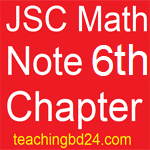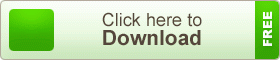# JSC Math Note 6th Chapter Simple Simultaneous Equation

JSC Math Note 6th Chapter Simple Simultaneous Equation. The role of the equation to solve mathematical problems is important. We learned to solve a simple equation in the sixth and seventh class and formulate the equation of this real problem. In the Seventh Class, we learned about the fiduciary rules of the equation, the rules of exclusion, the quantization rules, and symmetry rules. In addition, we learned how to solve the equation with the help of a chart. In this chapter, there are detailed discussions about the solutions with the help of solutions and graphs in different modes of simple variation of two variables.

## JSC Math Note 6th Chapter Simple Simultaneous Equation###(1) Replacement method

In this process we can solve the following steps:

(A) Expression of one of the variables from one of the two equations is expressed through the other.

(B) Fix a variable equation by setting the value of the variables obtained from the other equation.

(C) Determine the value of another variable by putting one of the two given equations in the given solution

(2) The removal process

In this process, following the steps can be solved:
(A) Both equations given must be multiplied by two numbers or numbers, as the coefficient of any one variable is equal.
(B) If the coefficient of a variable is the same and the same sign, then the subtraction of the equation should be subtracted, otherwise, it should be added. The quadrilateral (or sum) equation will be a single simple equation of a variable.

(C) To determine the value of the variable in the solution of simple equations.
(D) Determine the value of the other variables by putting any given equation in the given variable value

teachingbd24.com is such a website where you would get all kinds of necessary information regarding educational notes, suggestions and questions’ patterns of school, college, and madrasahs. Particularly you will get here special notes of physics that will be immensely useful to both students and teachers. The builder of the website is Mr. Md. Shah Jamal Who has been serving for 33 years as an Asst. Professor of BAF Shaheen College Dhaka. He expects that this website will meet up all the needs of Bengali version learners /students. He has requested both concerned students and teachers to spread this website home and abroad.